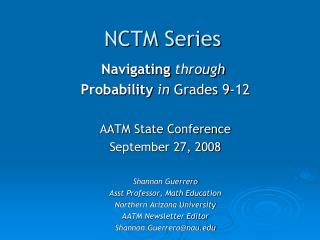Download PresentationNCTM Series

Loading in 2 Seconds...

# NCTM Series - PowerPoint PPT Presentation

NCTM Series. Navigating through Probability in Grades 9-12 AATM State Conference September 27, 2008 Shannon Guerrero Asst Professor, Math Education Northern Arizona University AATM Newsletter Editor Shannon.Guerrero@nau.edu. NCTM Navigation Series.I am the owner, or an agent authorized to act on behalf of the owner, of the copyrighted work described.
Download Presentation## NCTM Series

An Image/Link below is provided (as is) to download presentation

Download Policy: Content on the Website is provided to you AS IS for your information and personal use and may not be sold / licensed / shared on other websites without getting consent from its author.While downloading, if for some reason you are not able to download a presentation, the publisher may have deleted the file from their server.

- - - - - - - - - - - - - - - - - - - - - - - - - - E N D - - - - - - - - - - - - - - - - - - - - - - - - - -
Presentation Transcript
1. NCTM Series Navigatingthrough Probability in Grades 9-12 AATM State Conference September 27, 2008 Shannon Guerrero Asst Professor, Math Education Northern Arizona University AATM Newsletter Editor Shannon.Guerrero@nau.edu

2. NCTM Navigation Series • NCTM published series that offers activities, ideas & materials to roadmap implementation of Principles & Standards • Chapters organized around major concepts of particular NCTM content strand being targeted

3. NCTM Navigation Chapters • Each chapter begins with discussion of ensuing content & activities – provides big picture of NCTM strand & associated activities • Each activity consists of 3 elements – Engage, Explore, & Extend • Each component contains suggested materials, questions to pose, & teaching tips

4. Contents of the CD-ROM • Introduction • Table of Standards and Expectations, Number and Operations, Pre-K-12 • Applets • Binomial Distribution Simulator • Geometric Distribution Simulator • Random Number Generator • Adjustable Spinner • Blackline Masters and Templates • Readings from Publications of the National Council of Teachers of Mathematics and Other Sources

5. Why Study Probability? What are the “big idea” of probability for 9-12 grade students?

6. Why study probability? • Chance is all around us – we do not live in a totally deterministic world. • People must make decisions in the face of uncertainty. • Probability is indispensable for analyzing data; data are indispensable for estimating probabilities.

7. “Big Ideas” of Probability with High School Students • Probability as long-run relative frequency • Determining probability through an analysis of outcomes • Independence and conditional probability • Moving from sample spaces to probability distributions • Expected value as “average” behavior in the long run

8. Questions to PonderHow could we begin to answer? • What is the chance of an auto accident occurring at a complicated intersection? • What is the chance that a boy will develop hair loss if his father did? • What is the chance that a baseball player will get two hits in three consecutive batting attempts? • What is the chance that the stock market will go up three days in a row?

9. Chapter 1 – Probability as Long-Run Relative Frequency • Simulation can be useful tool for estimating probabilities • The cumulative stabilization of the relative frequency of an outcome in a large number of trials makes it a good estimate of the probability of the outcome.

10. Chapter Two – Sample Spaces • Students should be able to describe sample spaces such as the set of possible outcomes when four coins are tossed. • High school students should learn to identify mutually exclusive, joint, and conditional events. • All students should understand how to compute the probability of a compound event.

11. Chapter 3 – Independence and Conditional Probabilities • All students should understand the concepts of conditional probability and independent events. • Two outcomes are independent if the occurrence of one does no change the probability of the other. • Students can use the sample space to answer conditional probability questions.

12. Chapter 4 – Probability Models and Distributions • Students can gather simulated data to investigate the probability of an event. • Students can examine the declining probabilities of strings of outcomes from repeated trials. • All of the activities in this chapter direct students to run multiple trials with random numbers.

13. Chapter 5 – Expected Value • Expected value – the average value in the long run • All students should compute and interpret the expected value of random variables in simple cases. • Life insurance companies use expected-value calculations to determine premiums that they can expect to attract customers while ensuring a profit.